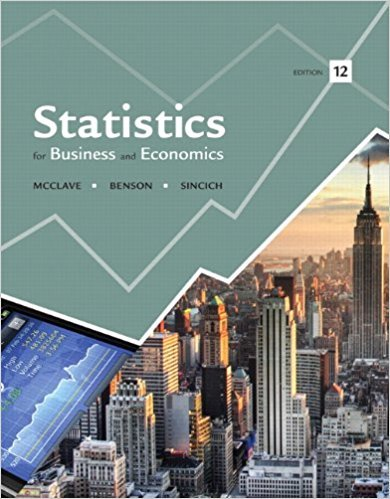×
Get Full Access to Statistics For Business And Economics - 12 Edition - Chapter 3 - Problem 2e
Get Full Access to Statistics For Business And Economics - 12 Edition - Chapter 3 - Problem 2e

×

# The diagram below describes the sample space of aISBN: 9780321826237 51

## Solution for problem 2E Chapter 3

Statistics for Business and Economics | 12th Edition

• Textbook Solutions
• 2901 Step-by-step solutions solved by professors and subject experts
• Get 24/7 help from StudySoup virtual teaching assistantsStatistics for Business and Economics | 12th Edition

4 5 1 374 Reviews
19
3
Problem 2E

Problem 2E

The diagram below describes the sample space of a particular experiment and events A and B.a. What is this type of diagram called?

b. Suppose the sample points are equally likely. Find P(A) and P(B).

c. Suppose P(1) = P(2) = P(3) = P(4) = P(5) = 1/20 and P(6) = P(7) = P(8) = P(9) = P(10) = 3/20. Find P(A) and P(B).

Step-by-Step Solution:

Solution

Step 1 of 3

a) We have to write the given diagram nameThe given diagram is called as venn diagram

Step 2 of 3

Step 3 of 3

##### ISBN: 9780321826237

Statistics for Business and Economics was written by and is associated to the ISBN: 9780321826237. The answer to “The diagram below describes the sample space of a particular experiment and events A and B. a. What is this type of diagram called?b. Suppose the sample points are equally likely. Find P(A) and P(B).c. Suppose P(1) = P(2) = P(3) = P(4) = P(5) = 1/20 and P(6) = P(7) = P(8) = P(9) = P(10) = 3/20. Find P(A) and P(B).” is broken down into a number of easy to follow steps, and 63 words. This textbook survival guide was created for the textbook: Statistics for Business and Economics , edition: 12. This full solution covers the following key subjects: Find, suppose, sample, diagram, below. This expansive textbook survival guide covers 15 chapters, and 1631 solutions. The full step-by-step solution to problem: 2E from chapter: 3 was answered by , our top Business solution expert on 07/21/17, 05:42AM. Since the solution to 2E from 3 chapter was answered, more than 596 students have viewed the full step-by-step answer.

Unlock Textbook Solution